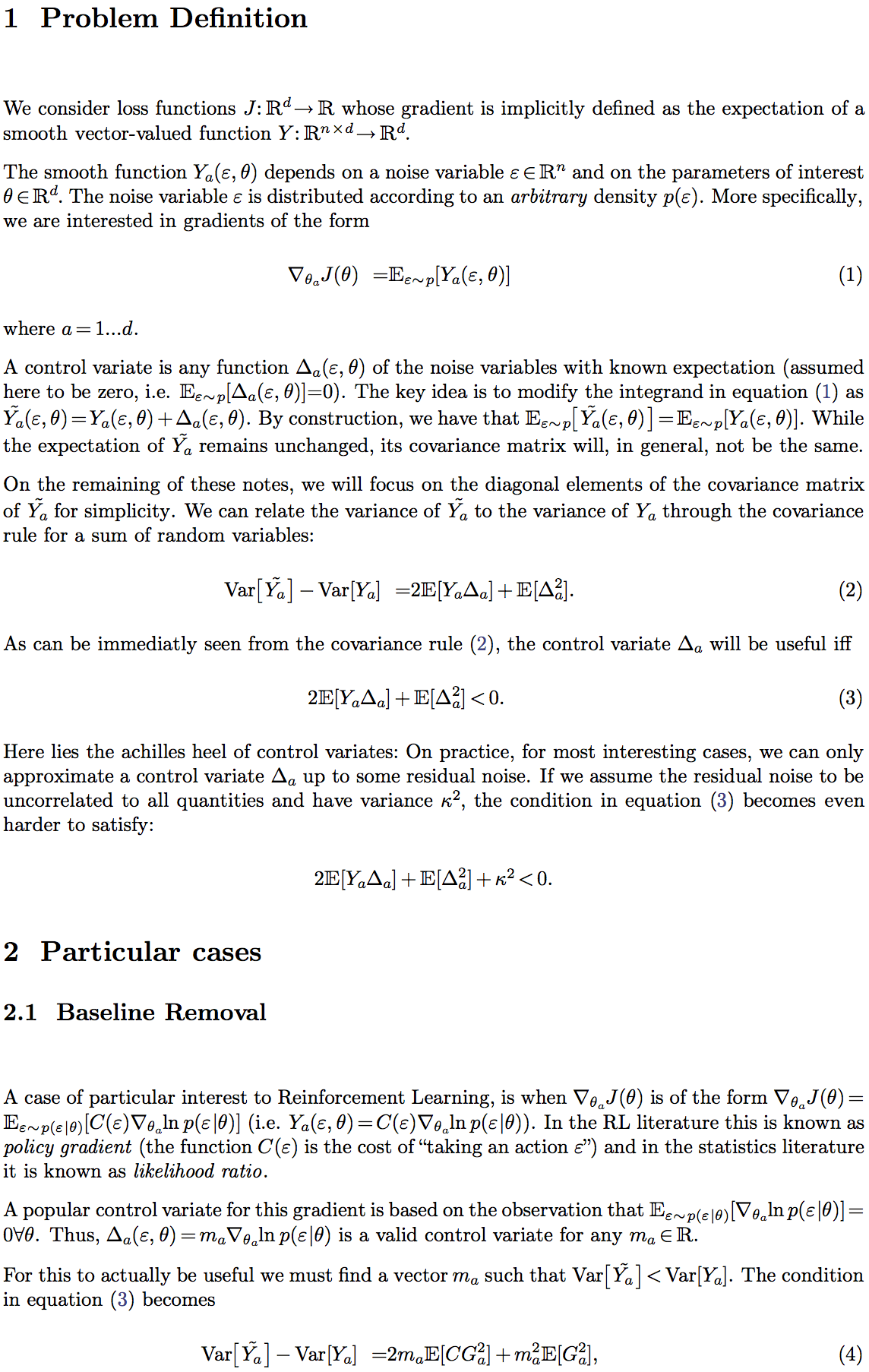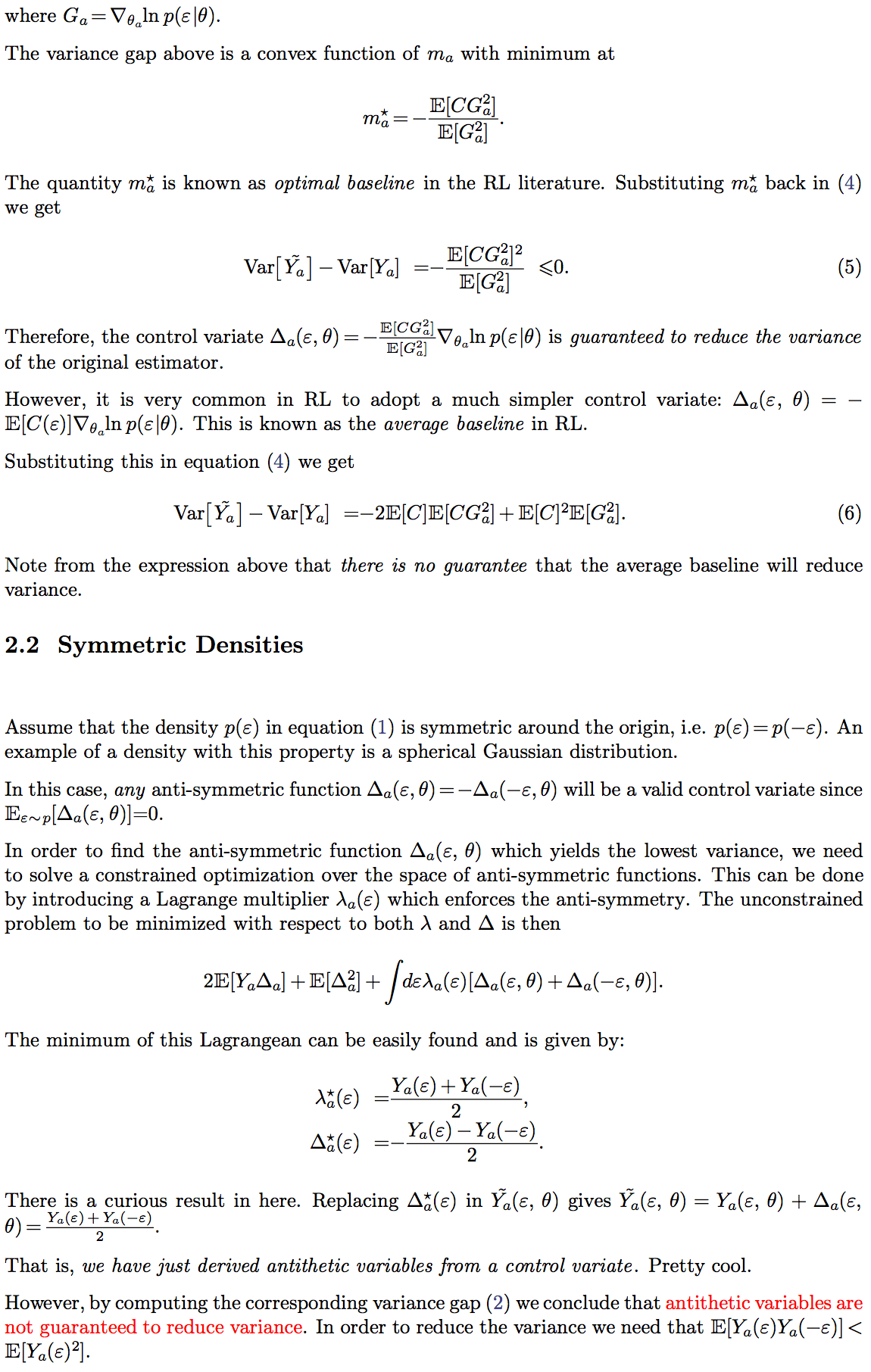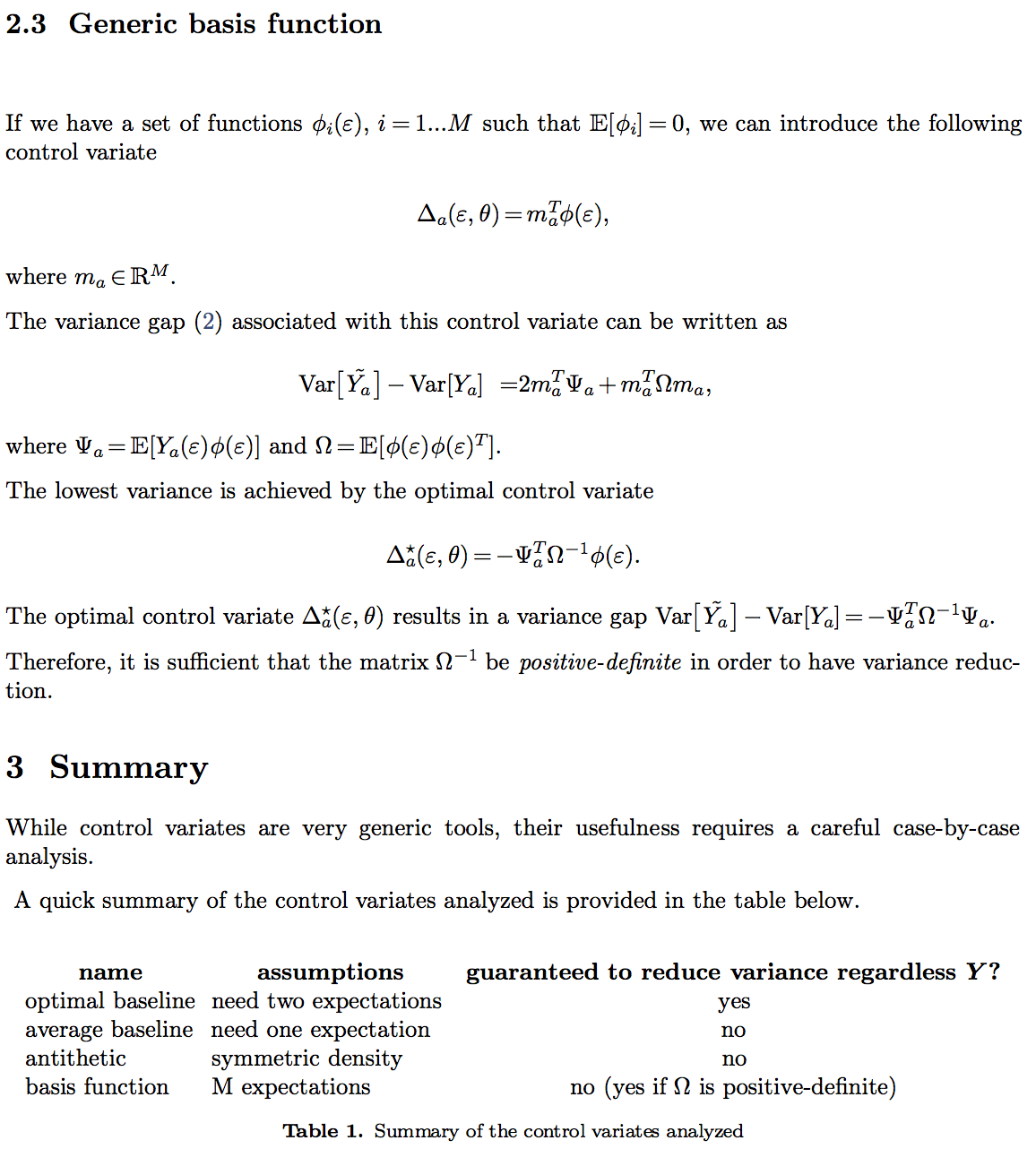# Motivation

For many problems in machine learning (ranging from Generative Models to Reinforcement Learning), we rely on Monte Carlo estimators of gradients for optimization. Often, the noise in our gradient estimators is a major nuisance factor affecting how close we can get to local optima.

There are many tricks around the corner to improve on this issue. A popular one is the “bias removal trick” widely known in the Reinforcement Learning literature.

Many of these tricks are particular cases of what is known as a control variate ( link1, link2, link3 ) a very generic method for variance reduction.

In this post I will try to characterize a few interesting and potentially useful applications of control variates and discuss their limitations.If you happen to know more interesting facts, theorems or use cases of control variates please let me know.

The pdf source of this post can be found here.

## 4 thoughts on “Useful Control Variates for Variance Reduction”

1.dohmatob on said:

There is a bug in the formula CG^2 ==> CG, yielding m = E(CG) / E(G^2). Also, the general solution (without assuming things are centered) is: cov(C, G) / var(G).

•DJR on said:

Hi, thank you but I think this formula is correct.
Var [(c (x) – m) G (x)] – Var [c (x) G (x)] = – 2 m E[c (x) G (x)^2] + m^2 E [G (x)^2]
The minimum of that with respect to m is m = E[c(x) G(x)^2]/E[G(x)^2].
Note that in the case of RL E[G(x)] = 0 by construction (policy gradient).

2.dohmatob on said:

Nice blogpost, BTW!

3.Jonathan on said:

Thanks for the post! I am trying to read the references you listed in paragraph 3, but link1 and link3 are broken. I wonder if you have those files?

This site uses Akismet to reduce spam. Learn how your comment data is processed.